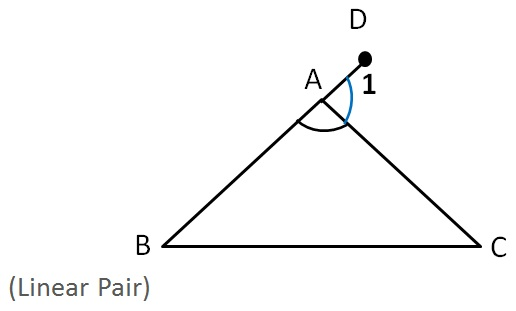Sum of Exterior Angles of Polygons

Let us consider a triangle ∆ ABC

Let ∠ 1 be the exterior angle∠ A + ∠1 = 180°      (Linear Pair)

∠ 1 = 180° − ∠ A

So, in a polygon

Interior angle + Exterior angle = 180°

Learn in your speed, with individual attention - Teachoo Maths 1-on-1 Class